# MA.5.NSO.2.2Export Print
Divide multi-digit whole numbers, up to five digits by two digits, including using a standard algorithm with procedural fluency. Represent remainders as fractions.

### Examples

The quotient 27÷7 gives 3 with remainder 6 which can be expressed as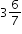.

### Clarifications

Clarification 1: Within this benchmark, the expectation is not to use simplest form for fractions.
General Information
Subject Area: Mathematics (B.E.S.T.)
Strand: Number Sense and Operations
Status: State Board Approved

## Benchmark Instructional Guide

• Equation
• Expression
• Whole Number

### Vertical Alignment

Previous Benchmarks

Next Benchmarks

### Purpose and Instructional Strategies

The purpose of this benchmark is for students to demonstrate procedural fluency while dividing multi-digit whole numbers with up to 5-digit dividends and 2-digit divisors. To demonstrate procedural fluency, students may choose the standard algorithm that works best for them and demonstrates their procedural fluency. A standard algorithm is a method that is efficient and accurate (MTR.3.1). In grade 4, students had experience dividing four-digit by one-digit numbers using a method of their choice with procedural reliability (MA.4.NSO.2.4). In grade 6, students will multiply and divide multi-digit numbers including decimals with fluency (MA.6.NSO.2.1).
• When students use a standard algorithm, they should be able to justify why it works conceptually. Teachers can expect students to demonstrate how their algorithm works, for example, by comparing it to another method for division (MTR.6.1).
• In this benchmark, students are to represent remainders as fractions. In the benchmark example, the quotient of 27 ÷ 7 is represented as 3 $\frac{\text{6}}{\text{7}}$. Students should gain understanding 6 represents $\frac{\text{6}}{\text{7}}$ of another group. Students are not expected to have mastery of converting that this quotient means that there are 3 full groups of 7 in 27, and the remainder of 6 between forms (fraction, decimal, percentage) until grade 6 but students should start to gain familiarity that fractions and decimals are numbers and can be equivalent (i.e., a remainder of $\frac{\text{1}}{\text{2}}$ is the same as 0.5). Writing remainders as fractions or decimals is acceptable. Similarly, students should be able to understand that a remainder of zero means that whole groups have been filled without any of the dividend remaining (MTR.5.1, MTR.7.1).
• Along with using a standard algorithm, students should estimate reasonable solutions before solving. Estimation helps students anticipate possible answers and evaluate whether their solutions make sense after solving.
• This benchmark supports students as they solve multi-step real-world problems involving combinations of operations with whole numbers (MA.5.AR.1.1). In a real-world problem, students should interpret remainders depending on its context.

### Common Misconceptions or Errors

• Students can make computational errors while using standard algorithms when they cannot reason why their algorithms work. In addition, they can struggle to determine where or why that computational mistake occurred because they did not estimate reasonable values for intermediate outcomes as well as for the final outcome. During instruction, teachers should expect students to justify their work while using their chosen algorithms and engage in error analysis activities to connect their understanding to the algorithm.

### Strategies to Support Tiered Instruction

• Instruction includes estimating reasonable values for quotients when dividing by two- digit divisors.
• For example, students make reasonable estimates for the quotient of 496 ÷ 24. Before using an algorithm, students can estimate the quotient to make sure that they are using the algorithm correctly and the answer is reasonable. Students can use multiples of 24 and their understanding of multiplication and division to estimate the quotient. Students may want their estimate to be as close to 496 as possible. So, knowing that 24 × 2 = 48, they can state that 24 × 20 = 480. A reasonable estimate for the quotient would be 20 because 480 is close to 496.”
• For example, students make reasonable estimates for the quotient of 94 ÷ 13. Explicit instruction could include stating, “Before using an algorithm, we will estimate the quotient to make sure that we are using the algorithm correctly and our answer is reasonable. The divisor of 13 is close to 10 and the dividend of 94 is close to 90. So, we can use 90 ÷ 10 = 9 to estimate that our quotient should be close to 9.”
• Instruction includes explaining and justifying mathematical reasoning while using a division algorithm to divide by two-digit divisors. Instruction also includes determining if an algorithm was used correctly by analyzing any errors made and reviewing the reasonableness of solutions.
• For example, the teacher connects place value with the partial quotients model to determine 496 ÷ 24. Students should not just view the digits as individual numbers but connect individual digits with the value of that number (e.g., 496 is 400 + 90 + 6). Instruction includes stating, “In this problem we are finding how many groups of 24 are in 496. We will subtract groups of 24 until we cannot subtract any more groups. The total number of groups that we can subtract is the quotient. We can subtract 10 groups of 24 two times, so the quotient is 20. We have a remainder of 16. The quotient is represented as 20 $\frac{\text{16}}{\text{24}}$ because we have 20 full groups of 24 in 496 and the remainder of 16 represents $\frac{\text{16}}{\text{24}}$ of another group.”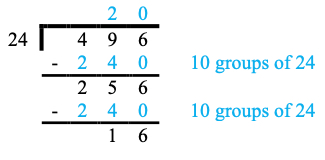• For example, connect place value with the partial quotients model to determine 94 ÷ 13. Students should not just view the digits as individual numbers but connect individual digits with the value of that number (e.g., 94 is 90 + 4). Instruction includes stating, “In this problem we are finding how many groups of 13 are in 94. We will subtract groups of 13 until we cannot subtract any more groups. The total number of groups that we can subtract is the quotient. We can subtract 7 groups of 13, so the quotient is 7. We have a remainder of 3. The quotient is represented as 7 $\frac{\text{3}}{\text{13}}$ because we have 7 full groups of 13 in 94 and the remainder of 3 represents $\frac{\text{3}}{\text{13}}$ of another group.”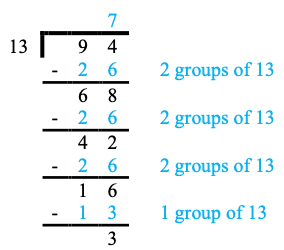• For example, students use an algorithm to solve 496 ÷ 24 and explain their thinking using place value understanding. Instruction includes stating, “In this problem we are finding how many groups of 24 are in 496. We will begin by dividing our largest place value first. Recognizing that the 4 represents 400, if your divide 400 by 24 the result will be less than 100, so the quotient won’t have any whole hundreds. Remember that 496 is the same as 49 tens 6 ones, so we will see how many groups of 24 are in 49 tens. We can also think of this as ___ × 24 = 49 tens. There are 20 groups of 24 in 49 tens, that’s 2 times 10 groups, so we can place a 2 in the tens place of the quotient. Next, we will subtract 49 tens – 48 tens (20 groups of 24 equal 48 tens) to find a difference of 1 ten. We can combine this 1 ten with the 6 ones remaining in 496. We now have 16 ones remaining from our original dividend of 496, this is not enough to make a group of 24. We have a remainder of 16. The quotient is represented as 20 $\frac{\text{16}}{\text{24}}$ because we have 20 full groups of 24 in 496 and the remainder of 16 represents $\frac{\text{16}}{\text{24}}$ of another group. Our quotient of 20 $\frac{\text{16}}{\text{24}}$ is close to our estimate of 24, this helps to determine that our answer is reasonable"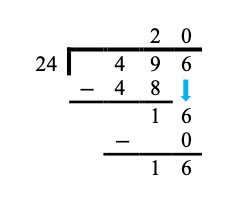• For example, students use an algorithm to solve 94 ÷ 13 and explain their thinking using base ten blocks and place value understanding. Instruction includes stating, “In this problem we are finding how many groups of 13 are in 94. We will begin by dividing our largest place value first. How many groups of 13 are in 9 tens? Recognizing that the 9 represents 90, if you divide 90 by 13 the result will be less than 10, so the quotient won’t have any whole tens. Remember that 94 is the same as 9 tens 4 ones and 94 ones, so we will see how many groups of 13 are in 94 ones. We can also think of this as ___ × 13 = 94. There are 7 groups of 13 in 94 ones. Next, we will subtract to find our remainder of 3. Our quotient is represented as 7 $\frac{\text{3}}{\text{13}}$ because we have 7 full groups of 13 in 94 and the remainder of 3 represents $\frac{\text{3}}{\text{13}}$ of another group. Our quotient of 7 $\frac{\text{3}}{\text{13}}$ is close to our estimate of 9, this helps us determine that our answer is reasonable.”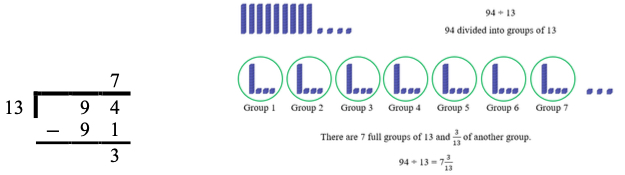• Instruction includes the use of place value columns to support place value understanding when using a division algorithm.
• Example: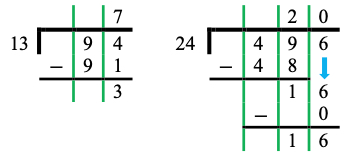The Magnolia Outreach organization is donating 6,924 pounds of rice to families in need. They pour all the rice into 15-pound containers.

• Part A. How many containers will they fill if they use all the rice?
• Part B. Will Magnolia Outreach be able to fill all the containers completely? If not, will the partially filled container be more or less than half-full? Explain how you know.

### Instructional Items

Instructional Item 1

What is the quotient of 498 ÷ 72?

*The strategies, tasks and items included in the B1G-M are examples and should not be considered comprehensive

## Related Courses

This benchmark is part of these courses.
5012070: Grade Five Mathematics (Specifically in versions: 2014 - 2015, 2015 - 2022, 2022 and beyond (current))
7712060: Access Mathematics Grade 5 (Specifically in versions: 2014 - 2015, 2015 - 2018, 2018 - 2022, 2022 and beyond (current))
5012065: Grade 4 Accelerated Mathematics (Specifically in versions: 2019 - 2022, 2022 and beyond (current))
5012015: Foundational Skills in Mathematics 3-5 (Specifically in versions: 2019 - 2022, 2022 and beyond (current))

## Related Access Points

Alternate version of this benchmark for students with significant cognitive disabilities.
MA.5.NSO.2.AP.2: Apply a strategy to divide two whole numbers up to two digits by one digit, including the possibility of whole number remainders.

## Related Resources

Vetted resources educators can use to teach the concepts and skills in this benchmark.

## Educational Software / Tool

Arithmetic Quiz:

In this activity, students solve arithmetic problems involving whole numbers, integers, addition, subtraction, multiplication, and division. This activity allows students to track their progress in learning how to perform arithmetic on whole numbers and integers. This activity includes supplemental materials, including background information about the topics covered, a description of how to use the application, and exploration questions for use with the java applet.

Type: Educational Software / Tool

## Formative Assessments

Students are asked to solve a division word problem with a two-digit divisor using a strategy based on place value.

Type: Formative Assessment

Analyzing and Applying Division:

Students are asked to analyze and explain another student’s division work in terms of a partial quotients strategy and to apply this strategy to another division problem.

Type: Formative Assessment

Dividing Using an Area Model With Larger Divisors:

Students are asked to interpret a division problem with a two-digit divisor that has been completed using an area model. If the student is successful, he or she is asked to determine the solution to a division problem with a two-digit divisor using an area model.

Type: Formative Assessment

Dividing Using Place Value With Larger Divisors:

Students are asked to complete a division problem using place value.

Type: Formative Assessment

Long Division - 1:

Students are asked to complete three long division problems (no remainders) to check for fluency with the standard division algorithm.

Type: Formative Assessment

Long Division - 3:

Students are asked to complete three long division problems (each with a remainder) to check for fluency with the standard division algorithm.

Type: Formative Assessment

Long Division - 2:

Students are asked to complete three long division problems (one with a remainder) to check for fluency with the standard long division algorithm.

Type: Formative Assessment

## Lesson Plans

Dividing for Equal Groups:

This lesson is meant to help solidify division understanding before teaching the standard algorithm.  Given a situational story, students will use base 10 blocks to model division in order to solve problems. It may be used for 4th or 5th grade depending on the size of the divisor.

Type: Lesson Plan

Make a Funny Partial Quotient Division Story:

This lesson introduces students to the area model and the partial quotient model to solve division problems with 2-digit divisors. Students are asked to apply both strategies to solve funny division problems that they help create by inserting information that is left blank in the story problems.

Type: Lesson Plan

Blessings in a Bag!!:

In this MEA, the students will help a charitable organization select 5 snack items from a list to provide nutritious snacks for children in low-income communities.  Students will practice using the four operations to solve real-world problems and use decimal notation to make calculations involving money.  Additionally, they will be asked to compare multi-digit numbers to the thousandths.

Type: Lesson Plan

Evan's Family Vacation:

Evan needs your help convincing his parents to rent a car for their family's vacation to Washington D.C. His parents are thinking of traveling in the family's old SUV that has no air and horrible gas mileage. Students will be asked to estimate each rental car's gas costs along with the weekly rental fee to rank the choices. In the twist, the students will be given safety information and must decide how to change their procedure with the new information.

Type: Lesson Plan

Easy as Pie Division!:

This is a hands-on lesson to show the relationship between division and multiplication. It provides a different method for solving long division that my students love.

Type: Lesson Plan

Diving deeper into division:

This lesson introduces students to dividing with 2 digit divisors.  Students are asked to apply strategies that they learned in dividing with 1 digit divisors such as partial quotients or breaking numbers apart using the distributive property.

Type: Lesson Plan

What Are They Thinking? Understanding Division:

This lesson uses a discovery approach to exploring the meaning of division. The students will utilize math practice standards as they analyze math solutions and explain their own solutions. Since the lesson analyzes division, it is a sound lesson to use to check student understanding before introducing efficient division algorithms.

Type: Lesson Plan

## Original Student Tutorial

Field Trip Frenzy (Part 4):

Learn when to write the remainder of a multi-step division process as a fraction or decimal in this interactive tutorial.

This is the final tutorial in the Field Trip Frenzy Series about remainders. Click below to open the other tutorials in this series.

Note: This tutorial extends beyond whole number quotients with whole number remainders to whole number quotients with fractional or decimal remainders.

Type: Original Student Tutorial

Minutes and Days:

This task requires division of multi-digit numbers in the context of changing units. In addition, the conversion problem requires two steps since 2011 minutes needs to be converted first to hours and minutes and then to days, hours, and minutes.

## Tutorial

Division: The importance of place value:

In this video tutorial from Khan Academy, learn about the importance of place value when dividing. The tutorial uses place value up to thousands to help students think about division.

Type: Tutorial

## STEM Lessons - Model Eliciting Activity

Blessings in a Bag!!:

In this MEA, the students will help a charitable organization select 5 snack items from a list to provide nutritious snacks for children in low-income communities.  Students will practice using the four operations to solve real-world problems and use decimal notation to make calculations involving money.  Additionally, they will be asked to compare multi-digit numbers to the thousandths.

Evan's Family Vacation:

Evan needs your help convincing his parents to rent a car for their family's vacation to Washington D.C. His parents are thinking of traveling in the family's old SUV that has no air and horrible gas mileage. Students will be asked to estimate each rental car's gas costs along with the weekly rental fee to rank the choices. In the twist, the students will be given safety information and must decide how to change their procedure with the new information.

## MFAS Formative Assessments

Analyzing and Applying Division:

Students are asked to analyze and explain another student’s division work in terms of a partial quotients strategy and to apply this strategy to another division problem.

Dividing Using an Area Model With Larger Divisors:

Students are asked to interpret a division problem with a two-digit divisor that has been completed using an area model. If the student is successful, he or she is asked to determine the solution to a division problem with a two-digit divisor using an area model.

Dividing Using Place Value With Larger Divisors:

Students are asked to complete a division problem using place value.

Students are asked to solve a division word problem with a two-digit divisor using a strategy based on place value.

Long Division - 1:

Students are asked to complete three long division problems (no remainders) to check for fluency with the standard division algorithm.

Long Division - 2:

Students are asked to complete three long division problems (one with a remainder) to check for fluency with the standard long division algorithm.

Long Division - 3:

Students are asked to complete three long division problems (each with a remainder) to check for fluency with the standard division algorithm.

## Original Student Tutorials Mathematics - Grades K-5

Field Trip Frenzy (Part 4):

Learn when to write the remainder of a multi-step division process as a fraction or decimal in this interactive tutorial.

This is the final tutorial in the Field Trip Frenzy Series about remainders. Click below to open the other tutorials in this series.

Note: This tutorial extends beyond whole number quotients with whole number remainders to whole number quotients with fractional or decimal remainders.

## Student Resources

Vetted resources students can use to learn the concepts and skills in this benchmark.

## Original Student Tutorial

Field Trip Frenzy (Part 4):

Learn when to write the remainder of a multi-step division process as a fraction or decimal in this interactive tutorial.

This is the final tutorial in the Field Trip Frenzy Series about remainders. Click below to open the other tutorials in this series.

Note: This tutorial extends beyond whole number quotients with whole number remainders to whole number quotients with fractional or decimal remainders.

Type: Original Student Tutorial

## Educational Software / Tool

Arithmetic Quiz:

In this activity, students solve arithmetic problems involving whole numbers, integers, addition, subtraction, multiplication, and division. This activity allows students to track their progress in learning how to perform arithmetic on whole numbers and integers. This activity includes supplemental materials, including background information about the topics covered, a description of how to use the application, and exploration questions for use with the java applet.

Type: Educational Software / Tool

Minutes and Days:

This task requires division of multi-digit numbers in the context of changing units. In addition, the conversion problem requires two steps since 2011 minutes needs to be converted first to hours and minutes and then to days, hours, and minutes.

## Tutorial

Division: The importance of place value:

In this video tutorial from Khan Academy, learn about the importance of place value when dividing. The tutorial uses place value up to thousands to help students think about division.

Type: Tutorial

## Parent Resources

Vetted resources caregivers can use to help students learn the concepts and skills in this benchmark.

Minutes and Days:

This task requires division of multi-digit numbers in the context of changing units. In addition, the conversion problem requires two steps since 2011 minutes needs to be converted first to hours and minutes and then to days, hours, and minutes.